GCSE Maths Probability

How To Calculate Probability

# How To Calculate Probability

Here we will learn how to calculate probability, including basic probability, mutually exclusive events, independent events and conditional probability.

There are also calculating probability worksheets based on Edexcel, AQA and OCR exam questions, along with further guidance on where to go next if you’re still stuck.

## What is probability?

Probability is the likelihood of an event occurring.

To find the probability of an event happening we use the formula

\text{Probability}=\frac{\text{number of desired outcomes}}{\text{total number of outcomes}}

For example,

Let’s look at the probability of getting an even number when a die is rolled.

The desired outcome is getting an even number. There are 3 even numbers on a die.

The total number of possible outcomes is 6 since there are 6 numbers on a die.

\text{The probability of getting an even number}=\frac{\text{number of desired outcomes}}{\text{total number of outcomes}}=\frac{3}{6}

Probabilities range from \bf{0} to \bf{1}.

If something has a probability of \bf{0} then it is impossible and if something has a probability of \bf{1} then it is certain.

We use the notation P(event) to represent the probability of an event happening.

For example,

If we wanted to write the probability of getting a 1 we could write P (1).

### What is probability?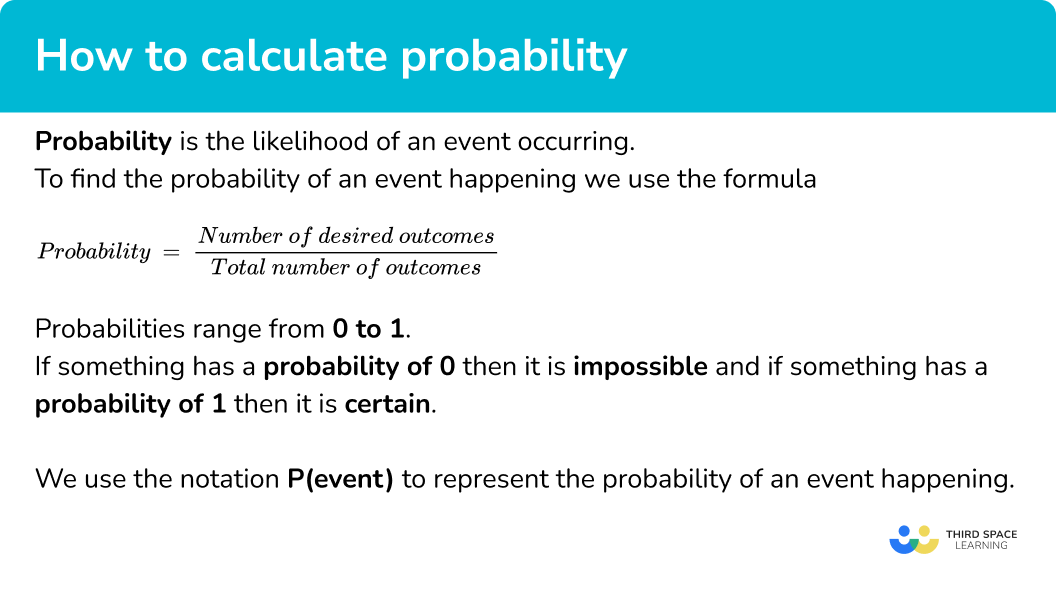### Calculating probabilities of combined events

Sometimes we want to find the probability of more than one event occurring. There are different probability rules that we can use.

• Mutually exclusive events

Mutually exclusive events are two or more events that cannot occur at the same time. For example, getting heads and tails when tossing a coin or rolling a 2 and a 3 on a die.

For mutually exclusive events: P(A or B) = P(A) + P(B)

If we have an exhaustive list of outcomes, their probabilities sum to 1. For example, the probability of getting an even or an odd number on a die.

The probability of getting an even number is \frac{3}{6}

and the probability of getting an odd number is \frac{3}{6}.

The probability of getting an even or an odd number is \frac{3}{6}+\frac{3}{6}=\frac{6}{6}=1.

Since getting an even number or an odd number covers all the possible outcomes, it is an exhaustive list and the probabilities add up to 1.

Step-by-step guide: Mutually exclusive events (coming soon)

• Independent events

Independent events are events which are not affected by the occurrence of other events. For example, if we roll a die twice, the outcome of the first roll and second roll have no effect on each other – they are independent events.

For independent events: P(A and B) = P(A) x P(B)

Step-by-step guide: Independent events (coming soon)

• Conditional probability

Conditional probability is the probability of an event occurring based on the occurrence of another event.

For conditional probability the probabilities are calculated based on what has already occurred.

For example, there are 5 counters in a bag, 2 are black and the rest are white.

A counter is picked at random and not replaced. A second counter is picked at random. The probability that the second counter is black depends on what the colour of the first counter was.

Step-by-step guide: Conditional probability

## How to calculate probability

In order to calculate probability:

1. Write out the basic probability
2. Solve the problem, using AND or OR rules as appropriate.

### Explain how to calculate probability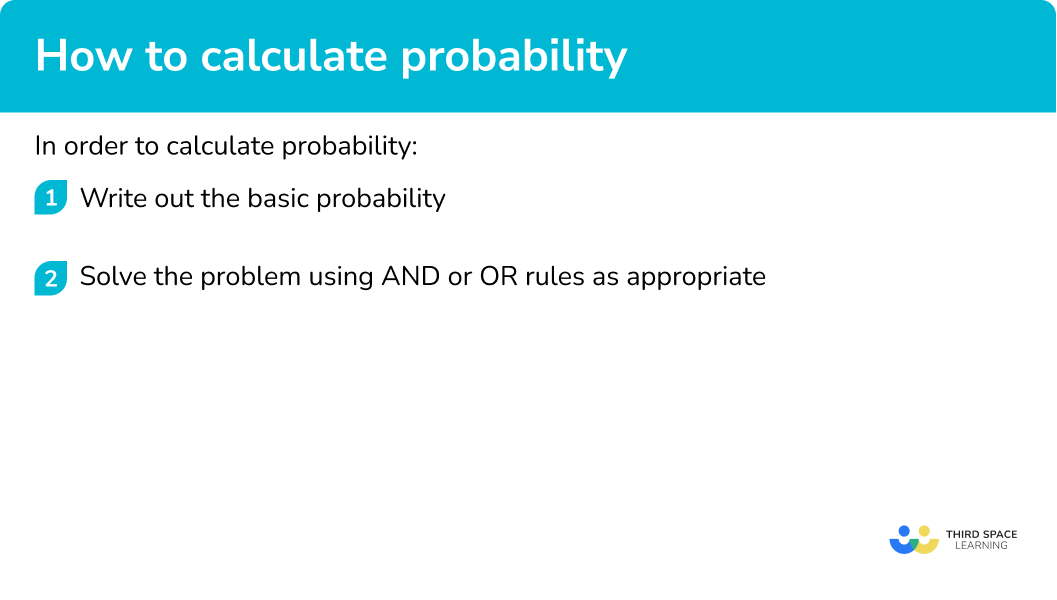## How to calculate probability examples

### Example 1: basic probability

Jamie has the following cards,

A card is chosen at random. Find the probability the card has a letter B on it.

1. Write out the basic probability.

We can write down the basic probability by using

\text{Probability}=\frac{\text{number of desired outcomes}}{\text{total number of outcomes}} .

The number of cards with B is 2, and the total number of cards is 11.

\text{Probability}=\frac{\text{number of desired outcomes}}{\text{total number of outcomes}}=\frac{2}{11}

2Solve the problem using AND or OR rules as appropriate.

Not needed as this is a basic probability question.

### Example 2: mutually exclusive events

What is the probability of landing on a 2 or a 3 on the following spinner?

We can write down the probability of getting a 2 and the probability of getting a 3.

P(2)=\frac{3}{8}

P(3)=\frac{2}{8}

\begin{aligned} \text{P(A or B)} &= \text{P(A)}+\text{P(B)}\\\\ \text{P(2 or 3)} &= \text{P(2)}+\text{P(3)}\\\\ \text{P(2 or 3)} &= \frac{3}{8}+\frac{2}{8}\\\\ &=\frac{5}{8} \end{aligned}

The probability of landing on a 2 or a 3 is \frac{5}{8}.

### Example 3: independent events

Olivia flips a coin and rolls a die. What is the probability that the coin lands on heads and the die lands on 1 ?

\text{P(1)}=\frac{1}{6}

\begin{aligned} \text{P(A and B)}&=\text{P(A)} \times \text{P(B)}\\\\ \text{P(Head and 1)}&=\text{P(Head)} \times \text{P(1)}\\\\ \text{P(Head and 1)}&=\frac{1}{2} \times \frac{1}{6} \\\\ &= \frac{1}{12} \end{aligned}

The probability that the coin lands on heads and the die lands on 1 is \frac{1}{12}.

### Example 4: using a tree diagram

The probability that Kate wins a game of tennis is 0.6. The probability that Billy wins a game of tennis is 0.7. Kate plays a match on Saturday and Billy plays a match on Sunday.

Find the probability that one of them wins and one of them loses.

We can work out the probabilities that the people do not win their tennis games.

\text{P(Kate NOT win)}=1-0.6=0.4

\text{P(Billy NOT win)}=1-0.7=0.3

For this question we are going to draw a tree diagram so we can clearly see the different outcomes.

For the outcome that one of them wins and the other loses, we could have Kate wins and Billy loses or Kate loses and Billy wins.

\text{P(Kate wins and Billy loses)}=0.6 \times 0.3=0.18

\text{P(Kate loses and Billy wins)}=0.4 \times 0.7=0.28

\text{P(one wins and one loses)}=0.18+0.28=0.46

The probability that one of them wins and the other loses is 0.46.

### Example 5: conditional probability

A bag contains 7 red marbles and 5 blue marbles. 1 marble is chosen at random.

The marble is red. A second marble is chosen. Find the probability the second marble is also red.

These are dependent events. The first event affects the probabilities for the second event.

\text{P(first marble is red)}=\frac{7}{12}

Given that one red marble has been chosen, there are now 6 red marbles and 11 marbles altogether. This is conditional probability.

\text{P(second marble is red)}=\frac{6}{11}

The probability that the second marble is red is \frac{6}{11}.

### Example 6: using a Venn diagram

The Venn diagram below shows the number of students who pass their mock exams in English and Maths.

A student is chosen at random. Given that the chosen student passes Maths, find the probability that they did not pass English.

This is a more complex probability question to work out. However we can work out how many students pass Maths.

12+9=21

This is a conditional probability question.

The desired outcome is that the student passes Maths but not English. There are 9 students who pass Maths but not English. The condition is that they pass Maths so we need to consider all the students who pass Maths. We know that 21 students pass Maths.

Therefore the probability that the student did not pass English given that they pass Maths is \frac{9}{21}.

### Example 7: using a two-way table

The two-way shows information about the gender and eye colour of the children in class 6.

A child is chosen at random. What is the probability that the child has green eyes given that they are male?

This is a more complex probability question to work out. However we can work out how many children are male.

2+9+4=15

This is a conditional probability question.

The desired outcome is that the child has green eyes but is male. There are 2 children who have green eyes and are male. The condition is that they are male, so we need to consider all the children who are male. We know that 15 children are male.

Therefore the probability that the child has green eyes given that they are male is \frac{2}{15}.

### Common misconceptions

For independent events P(A \ and \ B) = P(A) \times (P(B).

For mutually exclusive events P(A \ or \ B) = P(A) + P(B).

• Multiplying or dividing fractions incorrectly

To multiply fractions, multiply the numerators and multiply the denominators.

To divide fractions, turn the second one upside down and multiply.

Remember, fractions can only be added or subtracted if they have a common denominator.

• Not changing the probability for the second pick when picking two objects (conditional probability)

For example, if you have a bag containing 3 blue balls and 7 yellow balls, the probability of picking a blue ball on the first pick is \frac{3}{10} and the probability of picking a yellow ball on the first pick is \frac{7}{10}. The probabilities of picking the second ball depend on whether the first ball is replaced into the bag or not.

### Practice how to calculate probability questions

1. Luke has a deck of cards. Luke draws one card at random. Find the probability that Luke picks a King.

\frac{1}{13}\frac{1}{4}\frac{1}{52}\frac{4}{13}There are 4 Kings in a deck of cards. There are 52 cards in total.

\text{Probability}=\frac{4}{52}=\frac{1}{13}

2. The probability that Tom wears a certain colour t-shirt is shown below.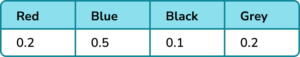Find the probability that Tom wears a black or a grey t-shirt.

0.20.150.30.7P(A \ or \ B) = P(A) + P(B)

P(black \ or \ grey) = 0.1+0.2 = 0.3

3. Evie takes the bus to work. The probability the bus is late on any given day is \frac{2}{5}, independent of whether it was late on the previous day. Find the probability the bus is late two days in a row.

\frac{2}{25}\frac{4}{25}\frac{4}{5}\frac{2}{25}P(A \ and \ B) = P(A) \times P(B)

P(late \ and \ late) =\frac{2}{5} \times \frac{2}{5} = \frac{4}{25}

4. Eddy has 10 red socks, 8 blue socks and 2 yellow socks.

Eddy picks a sock from the drawer. It is red.

Eddy picks a second sock. Find the probability it is also red.

\frac{9}{19}\frac{10}{20}\frac{9}{20}\frac{10}{19}Once Eddy has taken a red sock, there will be 9 red socks left and 19 socks left altogether. Therefore the probability is \frac{9}{19}.

5. The two-way table shows information about the height and hair colour of the children in class 3.A student is chosen at random. Find the probability the student has blonde hair given that they are under 120cm.

\frac{1}{3}\frac{6}{10}\frac{11}{24}\frac{1}{2}The desired outcome is a student that has blonde hair and is under 120cm. There are 6 such students.

The condition is that the student is under 120cm. There are a total of 12 students under 120cm.

The probability is \frac{6}{12}=\frac{1}{2} .

6. Rachel has 9 balls in a bag. 4 of the balls are blue and the other 5 are red. Rachel picks one ball, notes its colour and replaces the ball. She then picks a second ball. Find the probability the two balls she picks are the same colour.

\frac{16}{81}\frac{41}{81}\frac{25}{81}\frac{40}{81}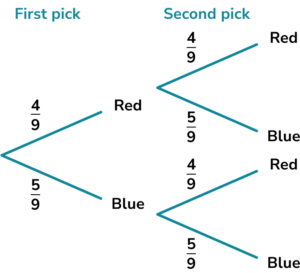Rachel can pick two red balls or two blue balls.

P(red \ and \ red) =\frac{4}{9} \times \frac{4}{9}=\frac{16}{81}

P(blue \ and \ blue) =\frac{5}{9} \times \frac{5}{9}=\frac{25}{81}

P(same \ colour) =\frac{16}{81}+\frac{25}{81}=\frac{41}{81}

The total probability is \frac{41}{81} .

### How to calculate probability GCSE questions

1. Jason picks one of the following cards at random.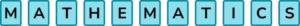(a) Find the probability that Jason picks a H.

(b) Find the probability that Jason picks an M or an A.

(c) Find the probability that Jason does not pick an M.

(3 marks)

(a)  \frac{1}{11}

(1)

(b)  \frac{2}{11}+\frac{2}{11}=\frac{4}{11}

(1)

(c)  \frac{9}{11}

(1)

2. (a) Yasmin designs a game where players must roll a dice and pick a card from a set of cards containing the numbers 1-10 once each. Players win if they roll a multiple of 3 and pick a card that is a multiple of 5.

Find the probability that a player wins the game.

(b) 150 people play the game.

Yasmin charges players £1 to play and winners receive a prize of £5. How much profit should Yasmin expect to make?

(6 marks)

(a)

\text{P(multiple of3)}=\frac{1}{3} \text{ or P(multiple of 5)} = \frac{1}{5}

(1)

\text{P(win)}=\frac{1}{3} \times \frac{1}{5} = \frac{1}{15}

(1)

(b)

150 \times £1=£150

(1)

Number of winners = \frac{1}{15} \times 150=10

(1)

10 \times £5=£50

(1)

Profit = £150-£50=£100

(1)

3. Janet has a bag of sweets containing three strawberry sweets, four blackcurrant sweets and five orange sweets.

Janet picks two sweets at random. Find the probability that both sweets are strawberry.

(3 marks)

\text{P(first sweet strawberry)}=\frac{3}{12}

(1)

\text{P(second sweet strawberry)}=\frac{2}{11}

(1)

\text{P(both strawberry)}=\frac{3}{12} \times \frac{2}{11}=\frac{6}{132} \ \text{or} \ \frac{1}{22}

(1)

## Learning checklist

You have now learned how to:

• Calculate basic probabilities
• Calculate the probability of two events happening
• Calculate the probability of mutually exclusive events
• Calculate the probability of independent events
• Deal with conditional probability
• Solve probability problems

## Still stuck?

Prepare your KS4 students for maths GCSEs success with Third Space Learning. Weekly online one to one GCSE maths revision lessons delivered by expert maths tutors.

Find out more about our GCSE maths tuition programme.